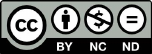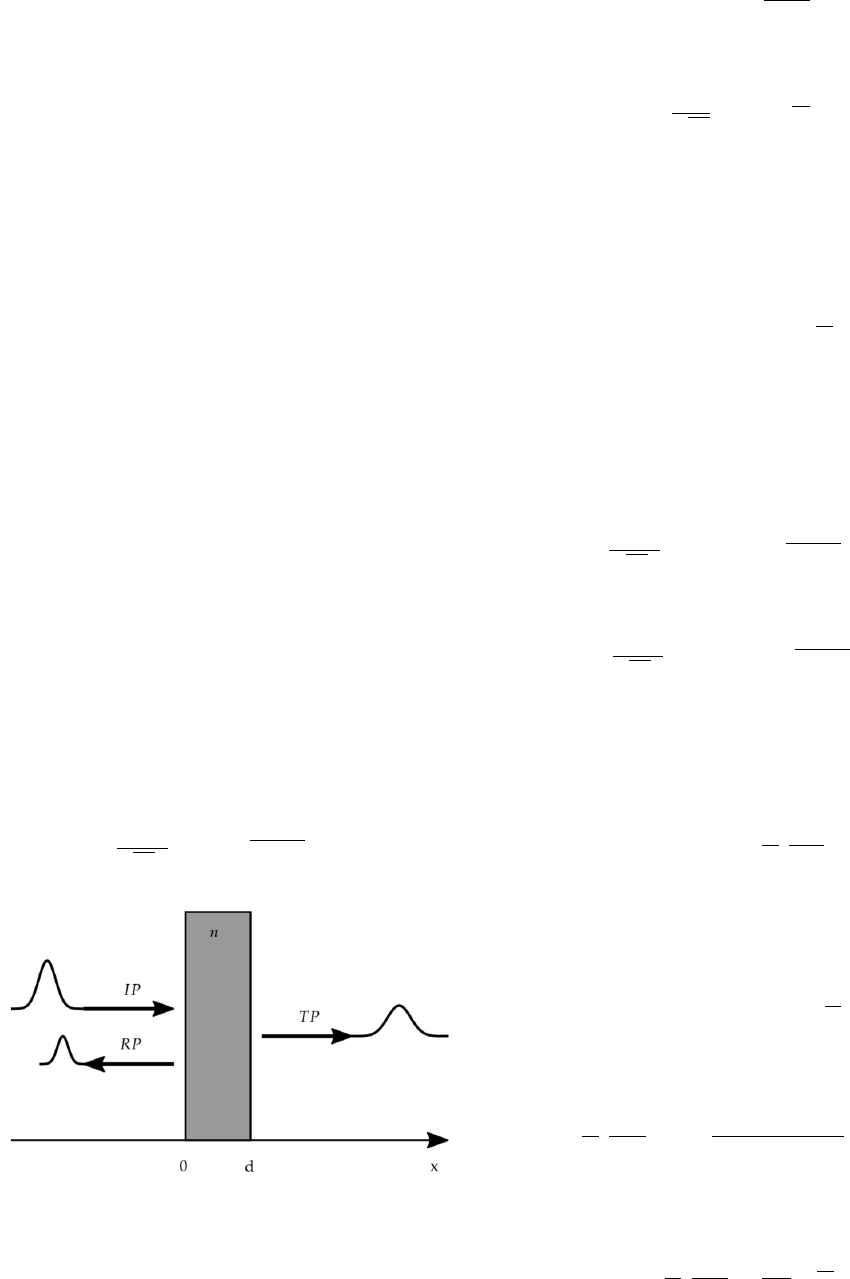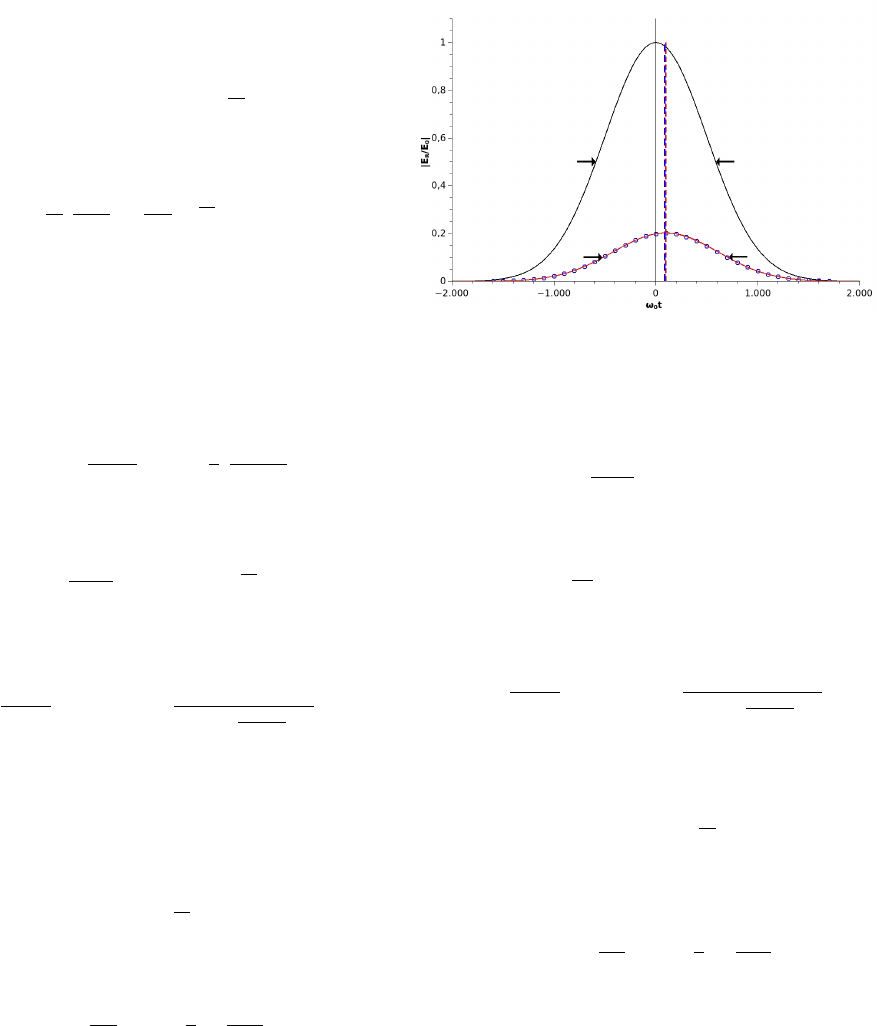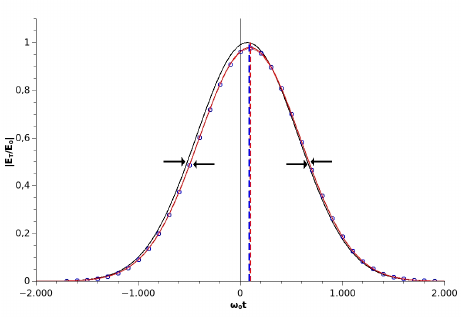Distortions of Gaussian pulses transmitted through
a transparent isotropic layer
Distorsi
´
on de pulsos gaussianos al atravesar una capa is
´
otropa
Eduardo O. Acosta
1
, Mar
´
ıa T. Garea
2
, Natalia C.
´
Alvarez
3
and Liliana I. Perez
4
Grupo de L
´
aser,
´
Optica de Materiales y Aplicaciones Electromagn
´
eticas
´
Paseo Col
´
on 850, C1063ACV, Buenos Aires, Argentina
1
eacosta@fi.uba.ar
2
mgarea@fi.uba.ar
Consejo Nacional de Investigaciones Cient
´
ıﬁcas y T
´
ecnicas (CONICET)
Buenos Aires, Argentina
3
nalvarez@fi.uba.ar
Universidad de Buenos Aires. Consejo Nacional de Investigaciones Cient
´
ıﬁcas y T
´
ecnicas
Instituto de Tecnolog
´
ıas y Ciencias de la Ingenier
´
ıa ”Hilario Fern
´
andez Long”
Paseo Col
´
on 850, C1063ACV, Buenos Aires, Argentina
4
lperez@fi.uba.ar
Abstract—Lasers can operate in two regimes: continuous-
wave mode or pulsed mode. In the simplest case, the former
mode corresponds to monochromatic beams with Gaussian
distribution of amplitudes (beam limited in space); whereas
the latter mode corresponds to polychromatic beams with
Gaussian distribution of frequencies (pulse limited in time).
When the pulsed beams are reﬂected and refracted in different
types of interfaces, they undergo peculiar distortions that
bear some parallelism with those found for beams limited in
space. These effects, as shown in a previous work, correspond
to time delay (ﬁrst order) and change of pulse width (second
order). The distortions are clearly limited by the principle of
causality and their interpretation, while not straightforward,
emerges clearly when the associated ﬁelds are expressed in
magnitude and phase. Since the analytical expressions are
not simple even for the case where the pulse is transmitted
through a single layer of linear, homogeneous, isotropic and
transparent material, it makes it difﬁcult to solve the inverse
problem. In this work, we present an alternative analytical
development that makes it possible to explicitly determine
these distortion effects when a pulse impinges normally
on a transparent isotropic layer immersed in a medium of
analogous characteristics.
Keywords: Gaussian pulses; Geometric Optics; Phase
shift
Resumen— Los l
´
aseres pueden operar en dos reg
´
ımenes:
modo continuo
´
o pulsado. En los casos m
´
as simples, el
modo continuo se corresponde a haces monocrom
´
aticos con
distribuci
´
on de amplitudes gaussiana (es un haz limitado en
el espacio); mientras que el modo pulsado corresponde a
haces policrom
´
aticos con distribuci
´
on gaussiana de frecuencias
reﬂejan y refractan en diferentes tipos de interfaces, sufren
distorsiones peculiares que tienen cierto paralelismo con los
como se muestra en un trabajo anterior, corresponden al
retardo de tiempo (primer orden) y al cambio de ancho de
pulso (segundo orden). Las distorsiones est
´
an claramente
´
on,
aunque no es directa, emerge claramente cuando los campos
asociados se expresan en magnitud y fase. Pero como las
expresiones anal
´
ıticas no son simples (incluso en el caso de que
el pulso se transmita a trav
´
es de una capa
´
unica de material
lineal, homog
´
eneo, isotr
´
opo y transparente) se hace dif
´
ıcil
resolver el problema inverso. En este trabajo, presentamos un
desarrollo anal
´
ıtico alternativo que hace posible determinar
expl
´
ıcitamente estos efectos de distorsi
´
on cuando un pulso
incide normalmente en una capa isotr
´
opica transparente
sumergida en un medio de caracter
´
ısticas an
´
alogas.
Palabras clave: Pulsos gaussianos;
´
Optica geom
´
etrica;
Cambio de fase
I. INTRODUCTION
When a 2D ﬁnite-width monochromatic beam undergoes
reﬂection and refraction at an planar interface between two
isotropic dielectric media, it is deformed. This deformation
is due to the fact that a ﬁnite beam of light is composed
of a superposition of a large number of plane waves that
propagate at slightly different angles of incidence with
respect to the central plane wave.
Under the paraxial condition, this leads to a small correc-
tion in the Fresnel equations, producing small effects that
modify the beam. The ﬁrst and second order effects are lat-
eral displacement or Goos-H
¨
anchen effect and angular shift
(ﬁrst order effects) and change of focal point and change
of width (second order effects). These have been studied
through different approaches as energy considerations 
, superposition of two or more waves with different
directions of propagation      or moment
theory  . Each of these methods have contributed
to the knowledge and determination of the different non-
geometrical effects.
Nevertheless, Gaussian or quasi-Gaussian beams have
been the most studied space-limited beams because they are
the lowest solution of the paraxial wave equation. In order
to obtain analytical expressions of these effects, usually the
Tamir’s generalized method (TGM) is used. This method,
an extension of the Tamir’s method , refers to the classic
Revista elektron, Vol. 2, No. 2, pp. 67-70 (2018)
ISSN 2525-0159
67model of multiple reﬂections on the interfaces of an isotropic
dielectric layer. The amplitudes of the successive terms of
the expansion rapidly decrease, i.e., it is a rapid convergence
method.
Chromatic dispersion is present in practically all optical
materials and is manifested as a dependence of the phase
velocity on the frequency or wavelength of light inclusive
in transparent media. As known, the group velocity is the
velocity that characterizes optical pulses either in dispersive
or non-dispersive media as well. An optical pulse propagates
at the group velocity
In the propagation, reﬂection and transmission of the real
Gaussian pulses there can be two types of deformations:
temporal and spatial. The latter corresponds to the distortion
that occurs with Gaussian beams (i.e. just spatially limited)
.
The propagation and transformation of laser pulses are
fundamental problems in the ﬁelds of laser technique, optical
communication, optical information processing, etc. Many
works have focused on propagation through dispersive and
anisotropic media   . However, there are no
studies about the distortion that occurs when a pulse (only
limited in time)is reﬂected or transmitted through non-
dispersive dielectric interfaces.
In this work we study the distorsions that occur on a
Gaussian pulse just limited in time when it is reﬂected
or transmitted on a single layer of non-dispersive material
using an analytical development alternative to TGM. The
alternative method applied to these type of pulses is a
generalization of the so call moment theory of light beam
propagation   . Finally the results obtained with
both methods are compared.
II. INCIDENT ELECTRIC FIELD OF A GAUSSIAN PULSE
Consider a Gaussian pulse, centered on a mean frequency
ω
0
and spectral width σ, which propagates in vacuum that
impinges normally on a parallel plate of refractive index n
and thickness d as shown in the ﬁgure 1. The electric ﬁelds
corresponding to the incident pulse
~
E
1
are given by
~
E
1
(x, t) =
~
E
0
2πσ
Z
+
−∞
e
(ωω
0
)
2
2σ
2
e
( x/ct)
(1)
Fig. 1. Diagram of the system under analysis: incident (IP), reﬂected (RP)
and transmitted pulses (TP)
where ω
0
corresponds to the mean frequency. Without loss
of generality we assume that the vector
~
E has a ˆy direction.
The integral of Eq. (1) is solved by taking = (ωω
0
)
and using the series expansion of e
iz
=
P
j=0
(i)
j
z
j
/j!,
replacing in Eq. (1) we obtain:
~
E
1
(x, t) =
~
E
0
e
0
(x/ct)
X
k=0
(i)
j
j!
σ
j
(t x/c)
j
Q
j
(2)
with
Q
j
=
1
2π
Z
+
−∞
e
2
2
j
d
The term Q
j
of the Eq. (2) is the central moment of
the standard normal distribution E(Ω
n
). By the symmetry
of the normal function, the terms of odd j are canceled and
the moments of j pairs are calculated following the equation
E(Ω
2k
) = (2k)!/2
k
k!. Replacing in Eq. (2), we obtain the
electric ﬁeld for the incident Gaussian pulse:
~
E
1
(x, t) =
~
E
0
e
0
(x/ct)
e
σ
2
2
(x/ct)
2
(3)
III. REFLECTED AND TRANSMITTED ELECTRIC FIELD OF
A GAUSSIAN PULSE
Assuming that the incident pulse impinges the surface
normally, the electric ﬁelds corresponding to the reﬂected
~
E
2
and transmitted
~
E
3
pulses are given by
~
E
2
(x, t) =
~
E
0
2πσ
Z
+
−∞
R(ω)e
(ωω
0
)
2
2σ
2
e
(x/c+t)
(4)
~
E
3
(x, t) =
~
E
0
2πσ
Z
+ inf
−∞
T (ω)e
(ωω
0
)
2
2σ
2
e
(x/ct)
(5)
where R(ω) and T (ω) are the reﬂection and transmission
coefﬁcients. An analytical expression of the ﬁelds can be ob-
tained by approximating these coefﬁcients by a polynomial
series around the average frequency ω
0
:
S(ω) = S(ω
0
) +
X
k=0
1
k!
k
S
ω
k
ω
0
ω
k
(6)
where S is R or T and ω = ω ω
0
. Replacing Eq. (6)
in Eq. (4) and using the same calculation procedure as for
the incident ﬁeld, we obtain the reﬂected electric ﬁeld
~
E
2
(x, t) =
~
E
0
e
0
(x/c+t)
R(ω
0
)e
σ
2
2
(x/c+t)
2
+ A
2
(7)
with
A
2
=
X
k=1
i
k
k!
k
R
ω
k
ω
0
X
j=0
(i)
j
(x/c + t)
j
j!
σ
k+j+1
E(Ω
j+k
)
using the expression of the moments we can obtain a
simpler expression for A
2
A
2
=
X
k=1
i
k
k!
k
R
ω
k
ω
0
k
t
k
e
σ
2
2
(x/c+t)
2
Revista elektron, Vol. 2, No. 2, pp. 67-70 (2018)
ISSN 2525-0159
68
http://elektron.fi.uba.arIn the same way, replacing Eq. (6) in Eq. (5) and using
the same procedure the transmitted ﬁeld is written as
~
E
3
(x, t) =
~
E
0
e
0
((xd)/ct)
T (ω
0
)e
σ
2
2
((xd)/c+t)
2
+ A
3
(8)
with
A
3
=
X
k=1
i
k
k!
k
R
ω
k
ω
0
k
t
k
e
σ
2
2
((xd)/ct)
2
IV. COMPARISON WITH THE RESULTS OBTAINED BY
MEANS OF TAMIRS GENERALIZED METHOD
In the Tamir’s generalized Method (TGM) the logarithm
of the reﬂection and transmission coefﬁcients can be re-
placed by their second order approximations around the
mean frequency 
ln S(ω) = ln S(ω
0
)+
ln S
ω
ω
0
ω +
1
2
2
ln S
ω
2
ω
0
ω
2
(9)
Introducing Eq. (9) in Eq. (4) and integrating we obtain
~
E
T GM
2
(x, t) =
~
E
0
R(ω)
σ
2
e
0
(x/c+t)
e
σ
2
2
2
(x/ct+τ
2
)
2
(10)
where we deﬁne
τ
2
= i
ln R
ω
ω
0
and σ
2
2
=
σ
2
1 σ
2
2
ln R
ω
2
ω
0
(11)
Eq. (10) show that the pulse suffers a ﬁrst order effect,
corresponding to a complex time delay τ
2
and a second order
effect, corresponding to a complex change of half-width σ
2
.
To compare both methods we truncate the series from Eq.
(7) to second order (k = 2)
~
E
2
(x, t) =
~
E
0
e
0
(x/c+t)
e
σ
2
2
(x/c+t)
2
P
2
(u
2
) (12)
with u
2
= σ(x/c + t) and
P
2
(u) = R(ω
0
)
R
ω
ω
0
u
1
2
σ
2
2
R
ω
2
ω
0
(u
2
1)
When comparing expressions Eq. (7) and Eq. (10) it is
not possible to determine if both expressions are similar.
Figure 2 shows incident pulse and reﬂected pulse calcu-
lated using equations (7) and (10) for ω
0
= 1.77×10
15
s
1
;
σ = ω
0
/500 assuming a plate of thickness d = 22πc/ω
0
and
refractive index n = 1.33; calculated for x = 0 as a function
of the dimensionless time ω
0
t. The curves calculated using
both approximations show a similar shift, for the case of
the TGM approximation the shift of ω
0
τ 91, while in the
presented development it is ω
0
τ 98. However, the half-
width of the half-height pulse () in both cases coincides
with ω
0
= 607 being 1.03 times the width of the initial
pulse.
The electric ﬁeld of the transmitted pulse calculated using
the TGM approximation is calculated in a similar way by
replacing equation Eq. (9) in Eq. (5)
Fig. 2. Incident pulse (black), reﬂected pulse using the method of moments
(red) and TGM () calculated at x = 0
~
E
T GM
3
(x, t) =
~
E
0
S(ω)
σ
3
e
0
((xd)/ct)
e
B(t)
(13)
with
B(t) =
σ
2
3
2
((x d)/c + t + τ
3
)
2
where we deﬁne
τ
3
= i
ln T
ω
ω
0
and σ
2
3
=
σ
2
1 σ
2
2
ln T
ω
2
ω
0
(14)
In order to compare both methods we developed the Eq.
(8) to second order
~
E
3
(x, t) =
~
E
0
e
0
((xd)/ct)
e
σ
2
2
((xd)/ct)
2
P
3
(u
3
)
(15)
with u
3
= σ((x d)/c t) and
P
3
(u) = T (ω
0
)
T
ω
ω
0
u
1
2
σ
2
2
T
ω
2
ω
0
(u
2
1)
In Fig. 3 shows the incident pulse and the transmitted
pulse calculated using equations (15) and (13) calculated
for x = d. In this case the curves calculated using both
approximations show a similar shift ω
0
τ 93, however the
pulse half-width in both cases coincides ω
0
= 588 being
the same as that of the initial pulse.
V. CONCLUSION
We show that Gaussian pulses, when reﬂected or trans-
mitted on a single layer, exhibit peculiar distortions. We
determine expressions for the ﬁrst and second order effects:
time delay and pulse width. The method used (method
of moments) is an alternative to the generalized Tamir’s
method and extended to pulses limited in time. Although
the distortions are very low at optical frequencies and begin
to be noticeable at frequencies of microwaves or less we
can see that the results obtained do not differ numerically
from each other. Nevertheless, from the method of moments
it is not simple to extract explicit expressions for such
Revista elektron, Vol. 2, No. 2, pp. 67-70 (2018)
ISSN 2525-0159
69
http://elektron.fi.uba.arFig. 3. Incident pulse (black), transmittes pulse using the method of
moments (red) and TGM () calculated at x = 0
deformations; but instead the TGM provides explicit expres-
sions, simple to calculate. In all cases the major analytical
difﬁculty is to calculate explicit expressions of the real and
imaginary parts of the ﬁelds (or of their module and phases)
when dealing with complex interfaces (several interfaces).
Although Tamir’s approximation is likely to be better than
the second order and can easily been extended up to fourth
order, the latter is easily extended to higher orders since a
recurrence equation has been obtained
ACKNOWLEDGMENT
This work was supported by the Universidad de
Buenos Aires under Grants UBACyT (2014-2017)
20020130100346BA, (2017- 2020) 20020160100042BA
and (2017-2020) 20020160100052BA. Also, a postdoctoral
grant from CONICET for one of the authors is gratefully
acknowledged.
REFERENCES
 J. Picht, About the vibration process that corresponds
to any (astigmatic) beam, Annalen der Physik, vol.
382, no. 16, pp. 785–882, 1925. [Online]. Available:
https://onlinelibrary.wiley.com/doi/abs/10.1002/andp.19253821602
 K. Yasumoto and Y. Oishi, A new evaluation of the goos–hanchen
shift and associated time delay, Journal of Applied Physics,
vol. 54, no. 5, pp. 2170–2176, 1983. [Online]. Available:
https://doi.org/10.1063/1.332395
 F. Noether, About the distribution of the energy
ﬂow in total reﬂection, Annalen der Physik, vol.
403, no. 2, pp. 141–146, 1931. [Online]. Available:
https://onlinelibrary.wiley.com/doi/abs/10.1002/andp.19314030203
 K. Artmann, “Calculation of the lateral displacement of
the totally reﬂected beam, Annalen der Physik, vol.
437, no. 1-2, pp. 87–102, 1948. [Online]. Available:
https://onlinelibrary.wiley.com/doi/abs/10.1002/andp.19484370108
 T. Tamir, “Nonspecular phenomena in beam ﬁelds reﬂected
by multilayered media, J. Opt. Soc. Am. A, vol. 3,
no. 4, pp. 558–565, Apr 1986. [Online]. Available:
http://josaa.osa.org/abstract.cfm?URI=josaa-3-4-558
 W. Nasalski, “Three-dimensional beam reﬂection at di-
electric interfaces, Optics Communications, vol.